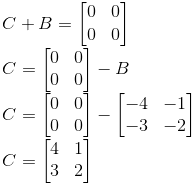# Selina Solutions Concise Maths Class 10 Chapter 9 Matrices Exercise 9(B)

Multiplication of a matrix by a scalar (real number) is the main concept discussed in this exercise. Students wishing to learn the correct steps of solving problems of the book can have a quick reference at the Selina Solutions for Class 10 Maths. All the solutions are prepared by subject experts at BYJU’S which is on par with the latest ICSE marking schemes. The solutions to the Concise Selina Solutions for Class 10 Maths Chapter 9 Matrices Exercise 9(B) are accessible in PDF format provided in the links given below.

## Selina Solutions Concise Maths Class 10 Chapter 9 Matrices Exercise 9(B) Download PDF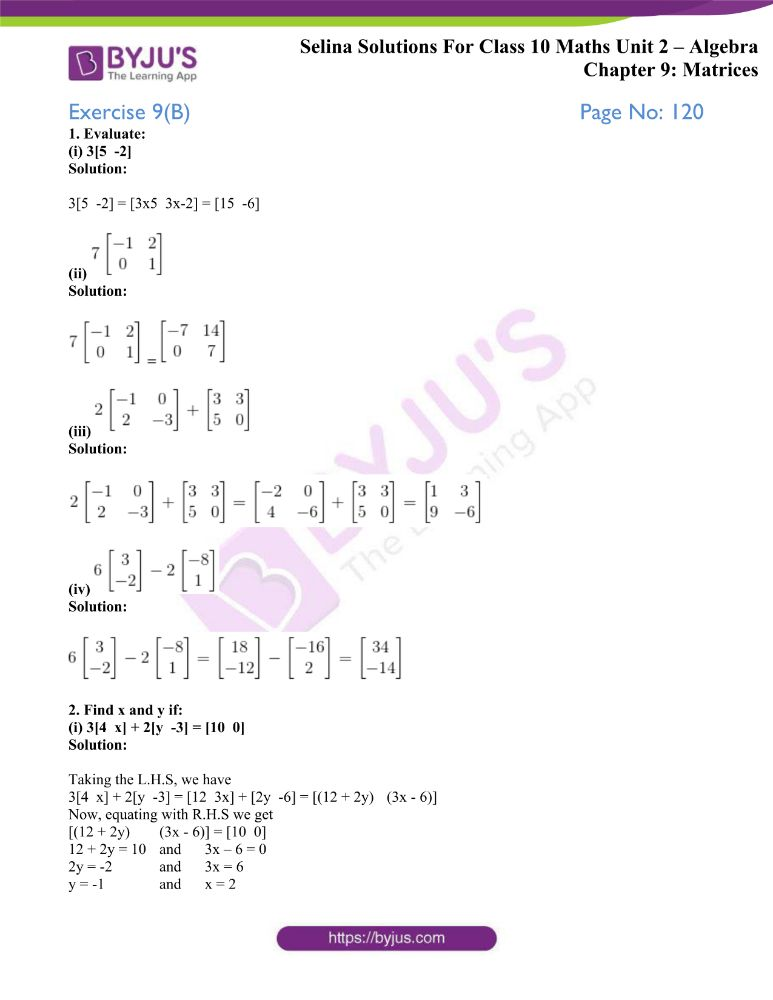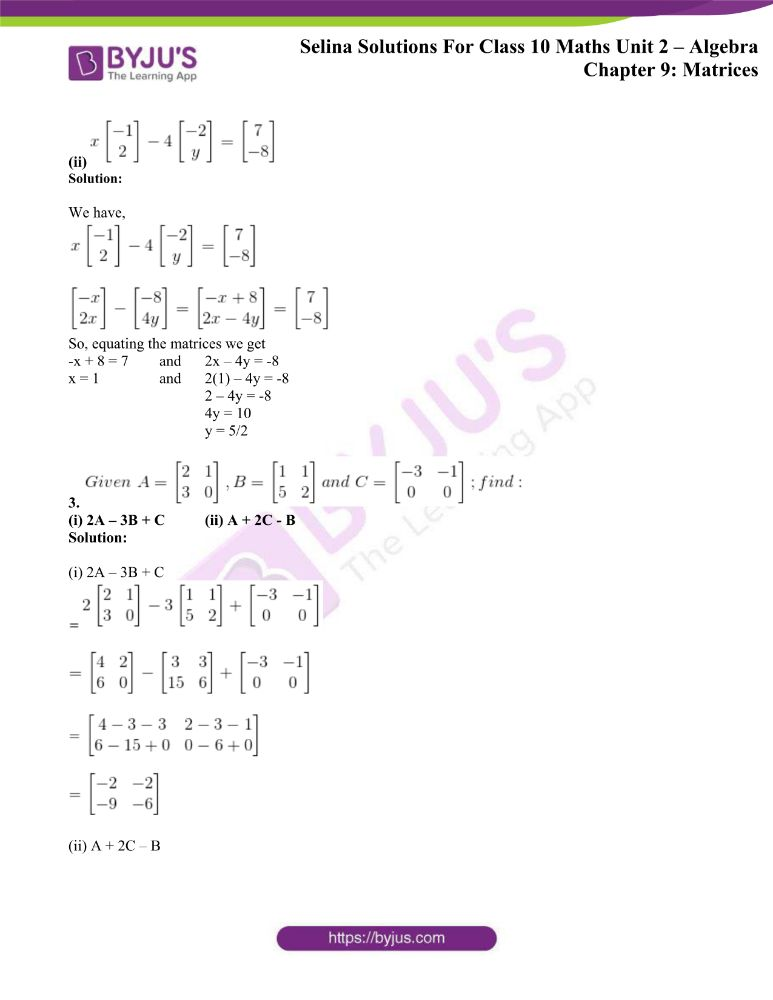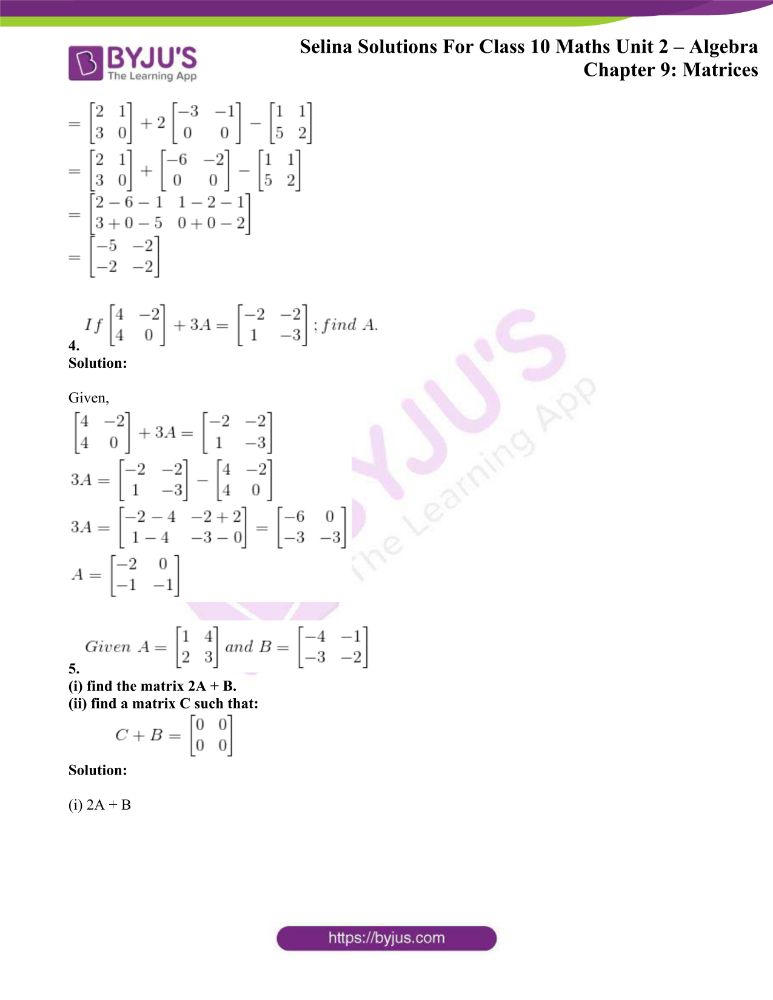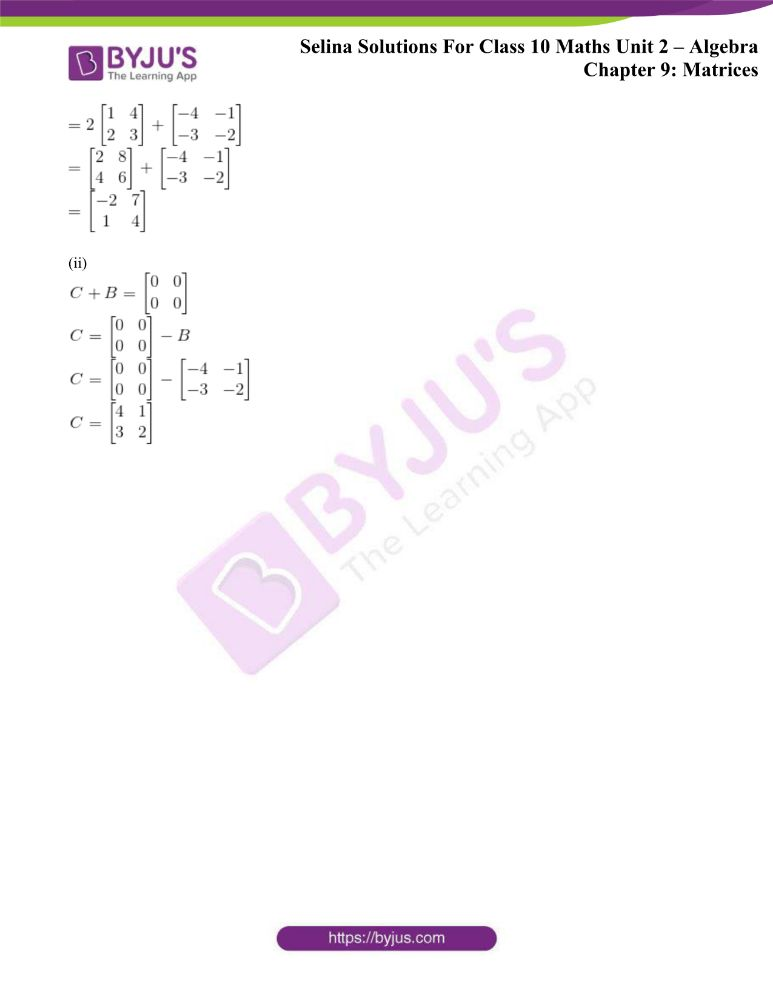### Access other exercises of Selina Solutions Concise Maths Class 10 Chapter 9 Matrices

Exercise 9(A) Solutions

Exercise 9(C) Solutions

Exercise 9(D) Solutions

## Access Selina Solutions Concise Maths Class 10 Chapter 9 Matrices Exercise 9(B)

1. Evaluate:

(i) 3[5 -2]

Solution:

3[5 -2] = [3×5 3x-2] = [15 -6]

(ii)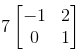Solution: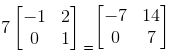(iii)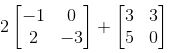Solution: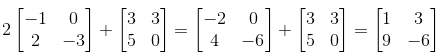(iv)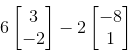Solution: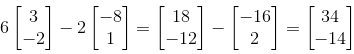2. Find x and y if:

(i) 3[4 x] + 2[y -3] = [10 0]

Solution:

Taking the L.H.S, we have

3[4 x] + 2[y -3] = [12 3x] + [2y -6] = [(12 + 2y) (3x – 6)]

Now, equating with R.H.S we get

[(12 + 2y) (3x – 6)] = [10 0]

12 + 2y = 10 and 3x – 6 = 0

2y = -2 and 3x = 6

y = -1 and x = 2

(ii)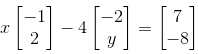Solution:

We have,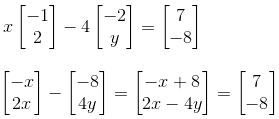So, equating the matrices we get

-x + 8 = 7 and 2x – 4y = -8

x = 1 and 2(1) – 4y = -8

2 – 4y = -8

4y = 10

y = 5/2

3.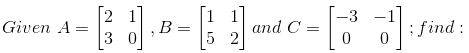(i) 2A – 3B + C (ii) A + 2C – B

Solution:

(i) 2A – 3B + C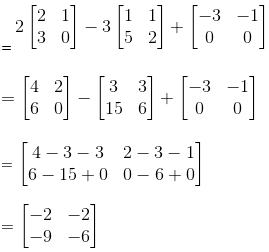(ii) A + 2C – B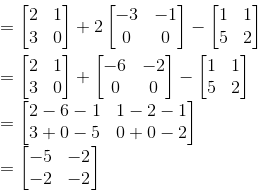4.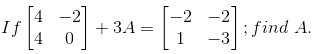Solution:

Given,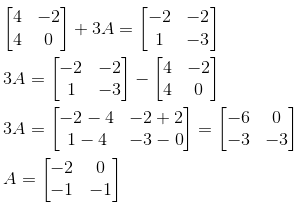5.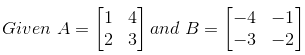(i) find the matrix 2A + B.

(ii) find a matrix C such that: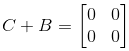Solution:

(i) 2A + B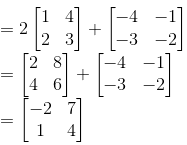(ii)